Скачать презентацию L 16 Interference At the end of

L 16 Waves interference.ppt

• Количество слайдов: 20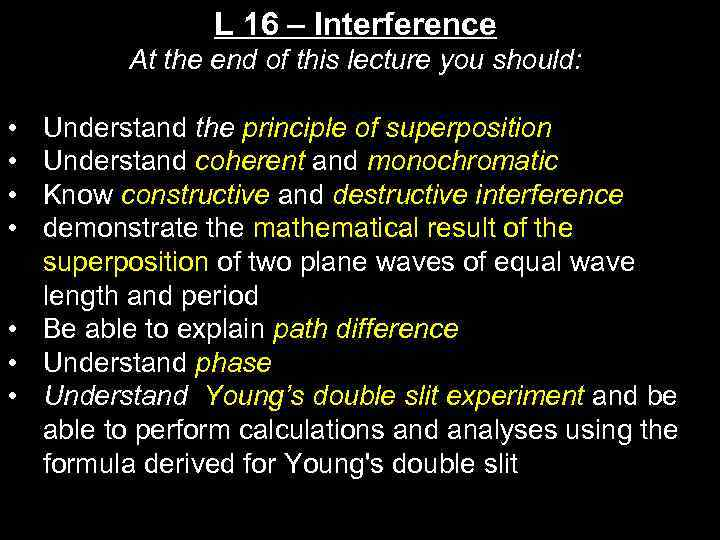L 16 – Interference At the end of this lecture you should: • • Understand the principle of superposition Understand coherent and monochromatic Know constructive and destructive interference demonstrate the mathematical result of the superposition of two plane waves of equal wave length and period • Be able to explain path difference • Understand phase • Understand Young’s double slit experiment and be able to perform calculations and analyses using the formula derived for Young's double slit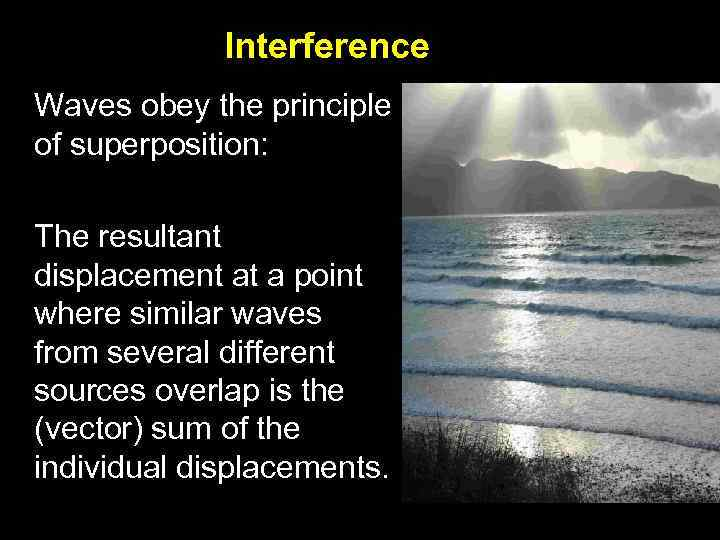Interference Waves obey the principle of superposition: The resultant displacement at a point where similar waves from several different sources overlap is the (vector) sum of the individual displacements.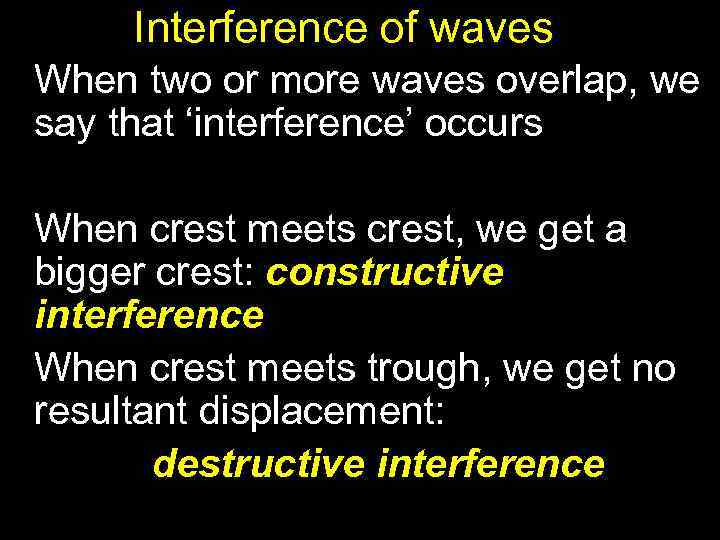Interference of waves When two or more waves overlap, we say that ‘interference’ occurs When crest meets crest, we get a bigger crest: constructive interference When crest meets trough, we get no resultant displacement: destructive interference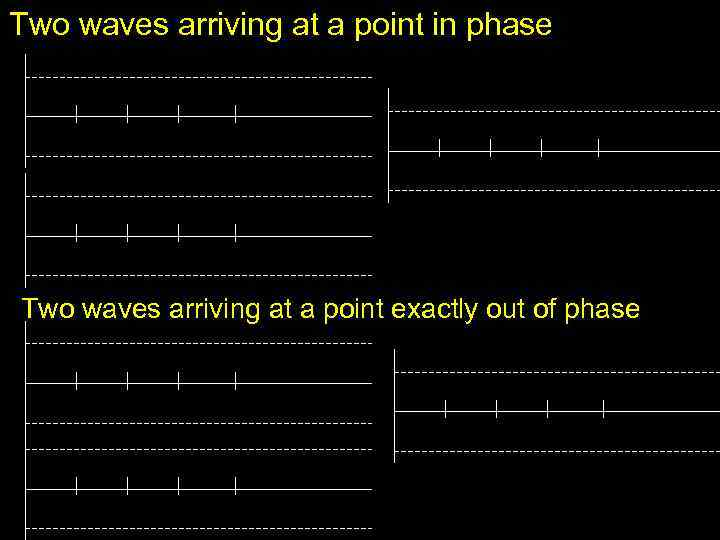Two waves arriving at a point in phase Two waves arriving at a point exactly out of phase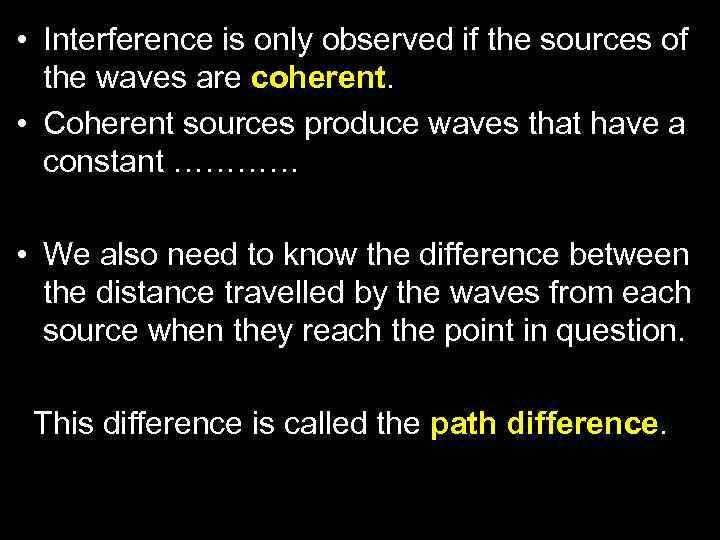• Interference is only observed if the sources of the waves are coherent. • Coherent sources produce waves that have a constant ………… • We also need to know the difference between the distance travelled by the waves from each source when they reach the point in question. This difference is called the path difference.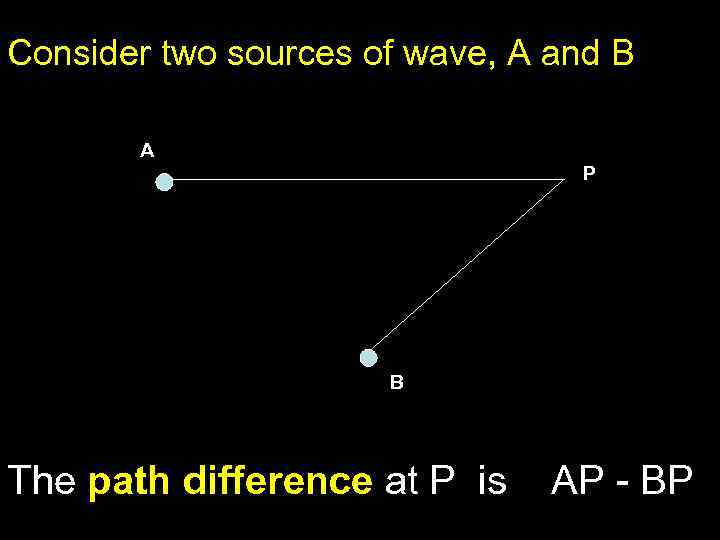Consider two sources of wave, A and B A P B The path difference at P is AP - BP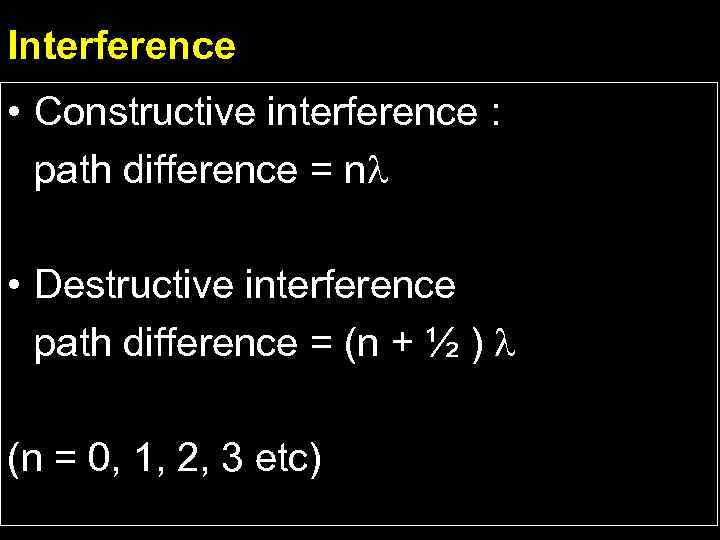Interference • Constructive interference : path difference = n • Destructive interference path difference = (n + ½ ) (n = 0, 1, 2, 3 etc)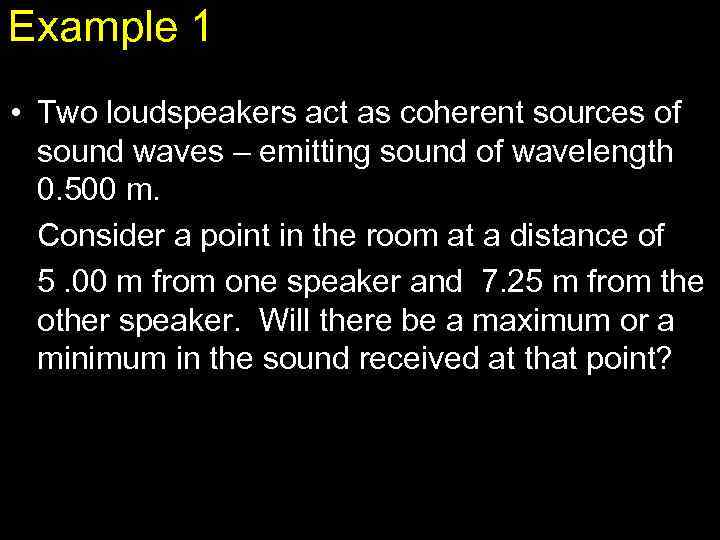Example 1 • Two loudspeakers act as coherent sources of sound waves – emitting sound of wavelength 0. 500 m. Consider a point in the room at a distance of 5. 00 m from one speaker and 7. 25 m from the other speaker. Will there be a maximum or a minimum in the sound received at that point?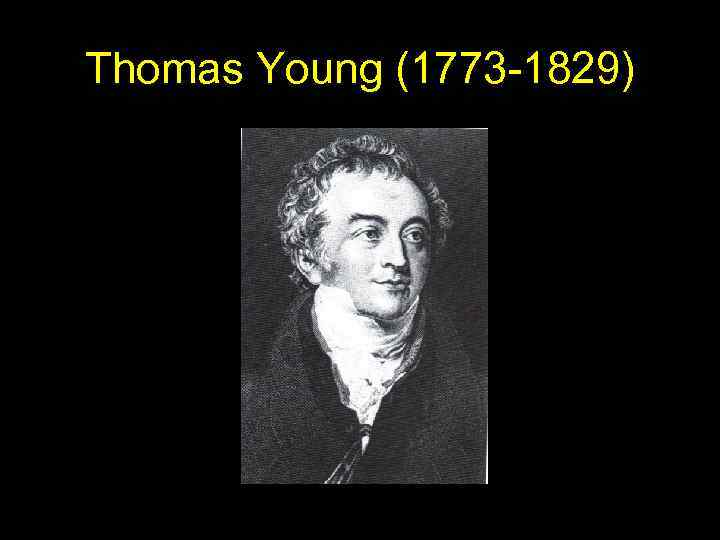Thomas Young (1773 -1829)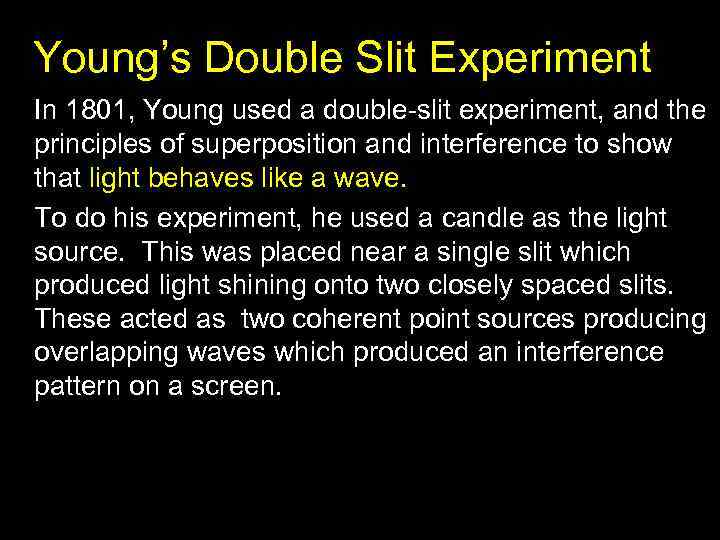Young’s Double Slit Experiment In 1801, Young used a double-slit experiment, and the principles of superposition and interference to show that light behaves like a wave. To do his experiment, he used a candle as the light source. This was placed near a single slit which produced light shining onto two closely spaced slits. These acted as two coherent point sources producing overlapping waves which produced an interference pattern on a screen.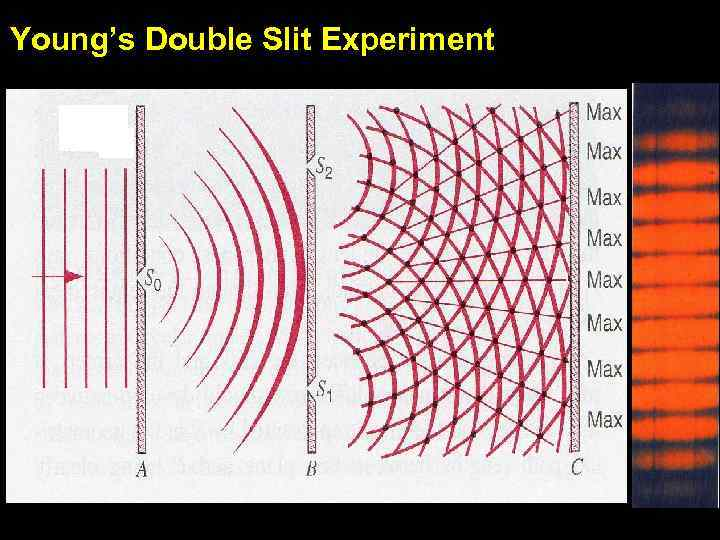Young’s Double Slit Experiment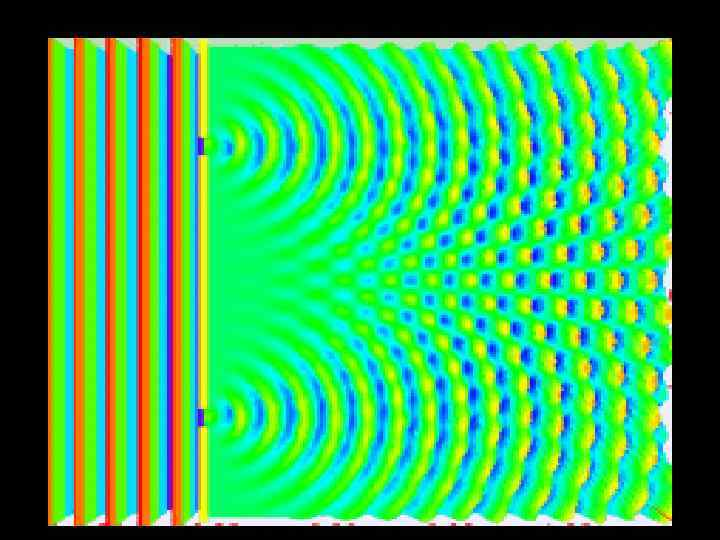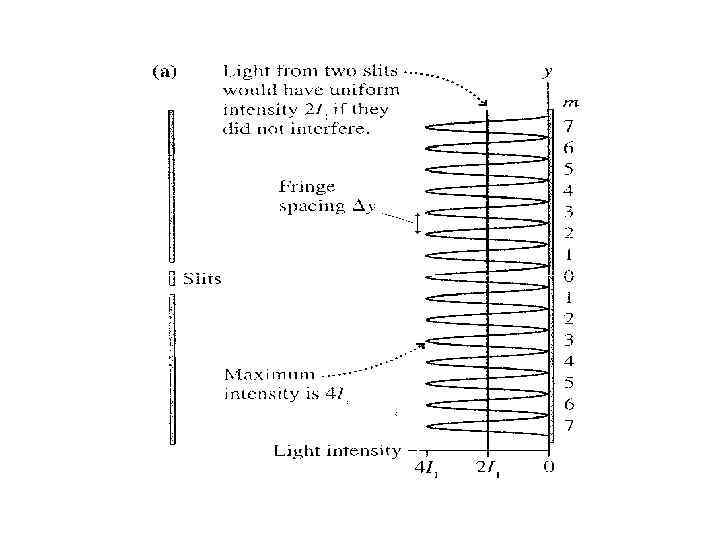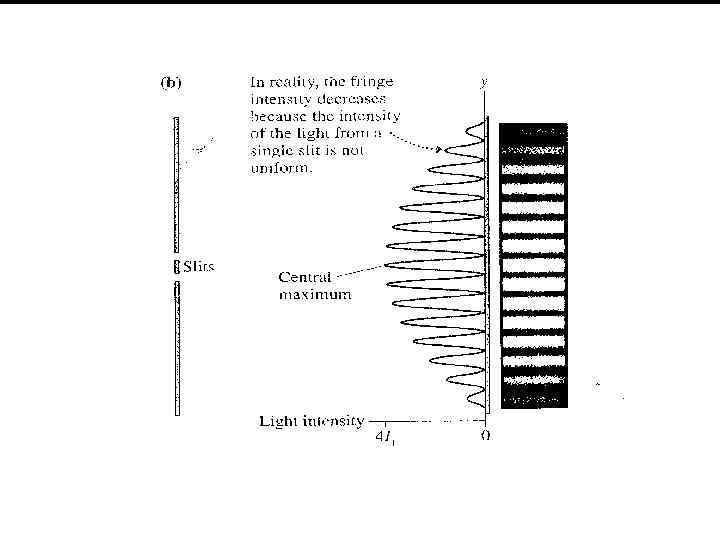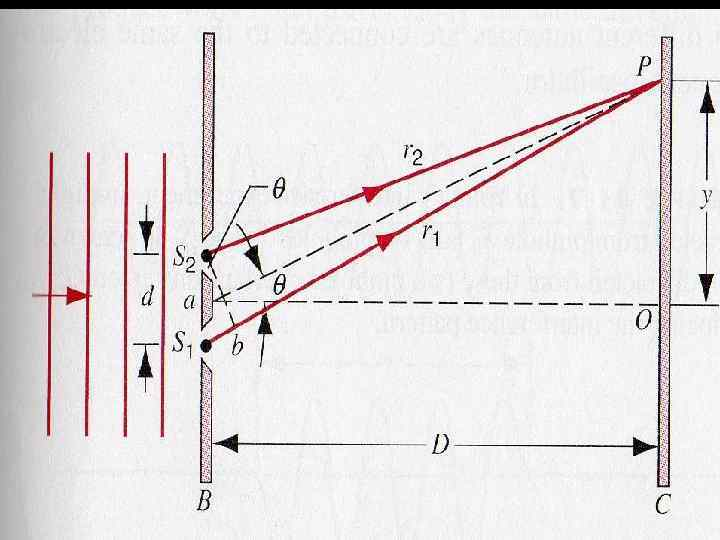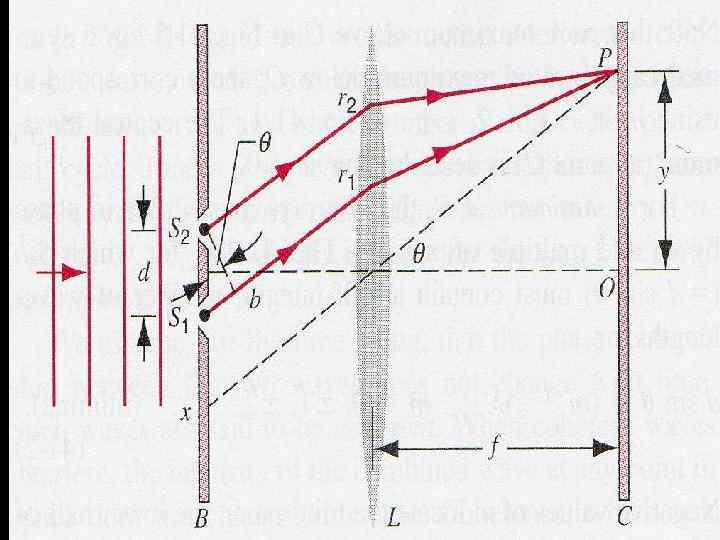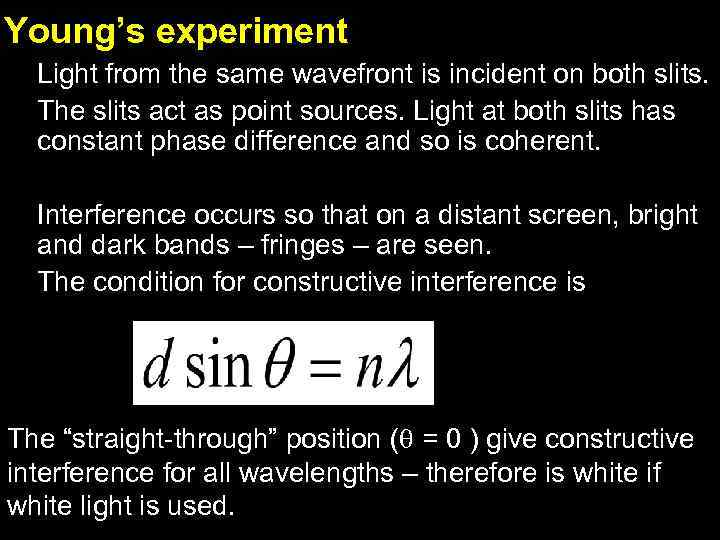Young’s experiment Light from the same wavefront is incident on both slits. The slits act as point sources. Light at both slits has constant phase difference and so is coherent. Interference occurs so that on a distant screen, bright and dark bands – fringes – are seen. The condition for constructive interference is The “straight-through” position ( = 0 ) give constructive interference for all wavelengths – therefore is white if white light is used.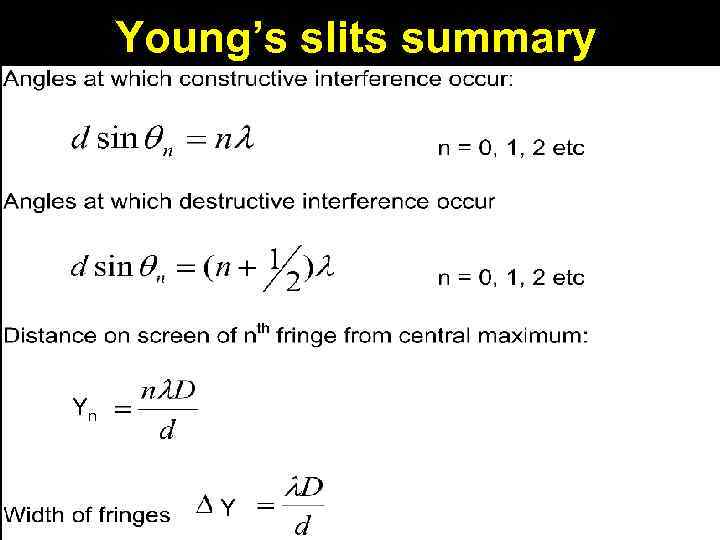Young’s slits summary Yn Y Y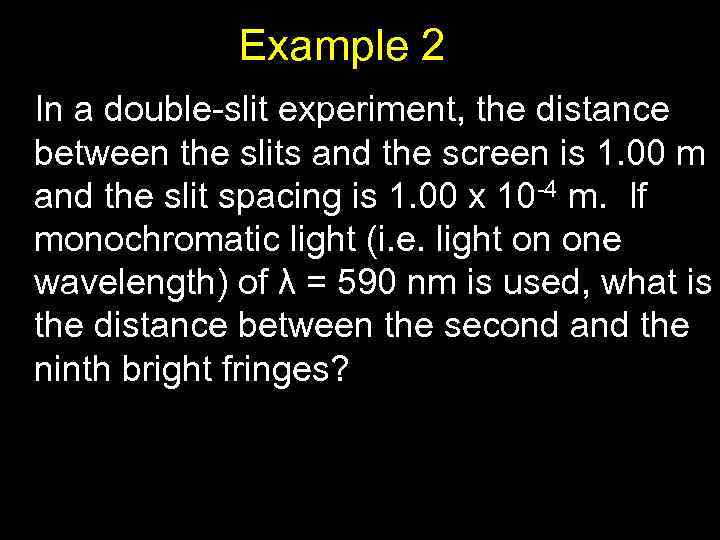Example 2 In a double-slit experiment, the distance between the slits and the screen is 1. 00 m and the slit spacing is 1. 00 x 10 -4 m. If monochromatic light (i. e. light on one wavelength) of λ = 590 nm is used, what is the distance between the second and the ninth bright fringes?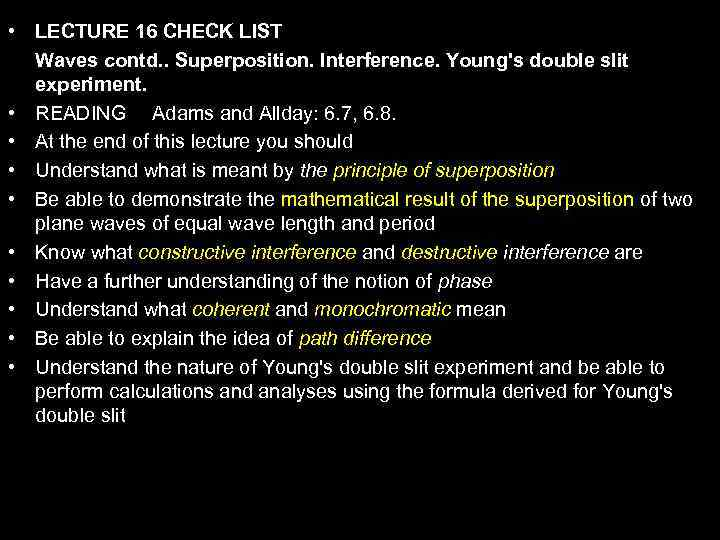• LECTURE 16 CHECK LIST Waves contd. . Superposition. Interference. Young's double slit experiment. • READING Adams and Allday: 6. 7, 6. 8. • At the end of this lecture you should • Understand what is meant by the principle of superposition • Be able to demonstrate the mathematical result of the superposition of two plane waves of equal wave length and period • Know what constructive interference and destructive interference are • Have a further understanding of the notion of phase • Understand what coherent and monochromatic mean • Be able to explain the idea of path difference • Understand the nature of Young's double slit experiment and be able to perform calculations and analyses using the formula derived for Young's double slit